# Absolute Value Functions

An absolute value function is a function that contains an algebraic expression within absolute value symbols. Recall that the absolute value of a number is its distance from $0$ on the number line.

The absolute value parent function, written as $f\left(x\right)=|\text{\hspace{0.17em}}x\text{\hspace{0.17em}}|$, is defined as

To graph an absolute value function, choose several values of $x$ and find some ordered pairs.

 $x$ $y=|\text{\hspace{0.17em}}x\text{\hspace{0.17em}}|$ −2 2 −1 1 0 0 1 1 2 2

Plot the points on a coordinate plane and connect them.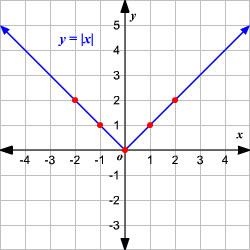Observe that the graph is V-shaped.

($1$) The vertex of the graph is $\left(0,0\right)$.

($2$) The axis of symmetry ($x=0$ or $y$-axis) is the line that divides the graph into two congruent halves.

($3$) The domain is the set of all real numbers.

($4$) The range is the set of all real numbers greater than or equal to $0$. That is, $y\ge 0$.

($5$) The $x$-intercept and the $y$-intercept are both $0$.

### Vertical Shift

To translate the absolute value function $f\left(x\right)=|\text{\hspace{0.17em}}x\text{\hspace{0.17em}}|$ vertically, you can use the function

$g\left(x\right)=f\left(x\right)+k$.

When $k>0$, the graph of $g\left(x\right)$ translated $k$ units up.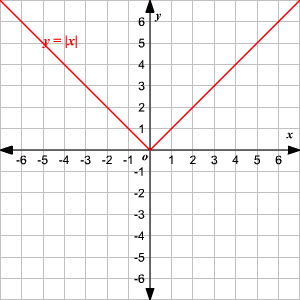When $k<0$, the graph of $g\left(x\right)$ translated $k$ units down.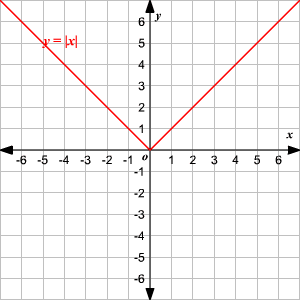### Horizontal Shift

To translate the absolute value function $f\left(x\right)=|\text{\hspace{0.17em}}x\text{\hspace{0.17em}}|$ horizontally, you can use the function

$g\left(x\right)=f\left(x-h\right)$.

When $h>0$, the graph of $f\left(x\right)$ is translated $h$ units to the right to get $g\left(x\right)$.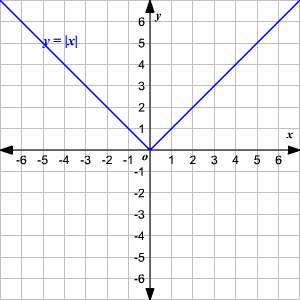When $h<0$, the graph of $f\left(x\right)$ is translated $h$ units to the left to get $g\left(x\right)$.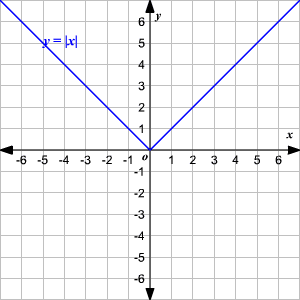## Stretch and Compression

The stretching or compressing of the absolute value function $y=|\text{\hspace{0.17em}}x\text{\hspace{0.17em}}|$ is defined by the function $y=a|\text{\hspace{0.17em}}x\text{\hspace{0.17em}}|$ where $a$ is a constant. The graph opens up if $a>0$ and opens down when $a<0$.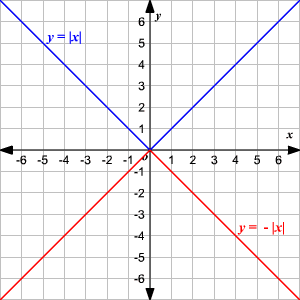For absolute value equations multiplied by a constant $\left(\text{for example,}y=a|\text{\hspace{0.17em}}x\text{\hspace{0.17em}}|\right)$,if $0, then the graph is compressed, and if $a>1$, it is stretched. Also, if a is negative, then the graph opens downward, instead of upwards as usual.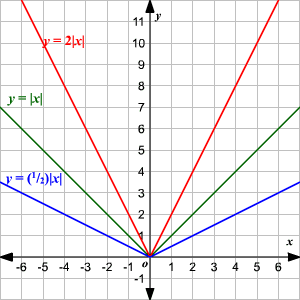More generally, the form of the equation for an absolute value function is $y=a|\text{\hspace{0.17em}}x-h\text{\hspace{0.17em}}|+k$. Also:

• The vertex of the graph is $\left(h,k\right)$.
• The domain of the graph is set of all real numbers and the range is $y\ge k$ when $a>0$.
• The domain of the graph is set of all real numbers and the range is $y\le k$ when $a<0$.
• The axis of symmetry is $x=h$.
• It opens up if $a>0$ and opens down if $a<0$.
• The graph $y=|\text{\hspace{0.17em}}x\text{\hspace{0.17em}}|$  can be translated $h$ units horizontally and $k$ units vertically to get the graph of $y=a|\text{\hspace{0.17em}}x-h\text{\hspace{0.17em}}|+k$.
• The graph $y=a|\text{\hspace{0.17em}}x\text{\hspace{0.17em}}|$ is wider than the graph of $y=|\text{\hspace{0.17em}}x\text{\hspace{0.17em}}|$ if $|\text{\hspace{0.17em}}a\text{\hspace{0.17em}}|<1$ and narrower if $|a|>1$.